# Item Sale Report in SQL

just for learning sake i want to create item summary report via sql in v4.

``````   SELECT  [MenuItemName],CONVERT(INT,SUM([Quantity])) as Qty,[Price],CONVERT(INT,[Price]*(SUM([Quantity]))) as TAmt

FROM [Orders]

WHERE [CreatedDateTime] > '{Start}' AND [CreatedDateTime] < '{End}' AND DecreaseInventory = 1 AND CalculatePrice <> 0

these two query are running but they are not tallying with my pivot report

select SUM(GT) FROM
(SELECT CONVERT(INT,[Price]*(SUM([Quantity]))) as GT
FROM [Orders]
WHERE [CreatedDateTime] > ‘{Start}’ AND [CreatedDateTime] < ‘{End}’ AND DecreaseInventory = 1 AND CalculatePrice <> 0

1 Like

Sorry, do you have a question here? What are your expected results? What is the results of the Pivot Report? Is it correct? Are you trying to match the results in SQL?

using the two query above i am trying to achieve a report equivalent to this:

``````[!Item Sale per Group:1,1,1,1:0]
``````

including summary/grand total for O.Quantity,O.Total

@madiha It’s not SQL but this does the same thing using Report Tags and no Pivot Table required:

``````[Item Sale per Group:1,1,1,1]
>||Grand Total: {REPORT ORDER DETAILS:O.Quantity.Sum}|Grand Total: \${REPORT ORDER DETAILS:O.Total.Sum}
``````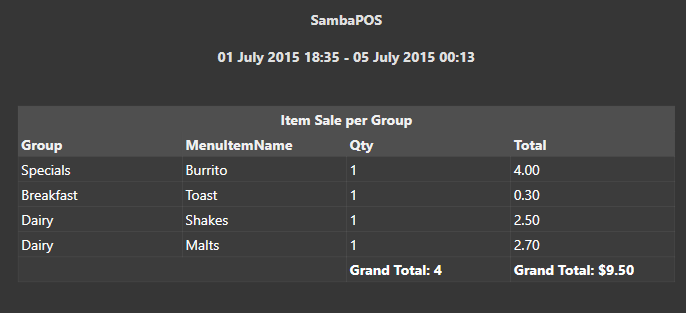@kendash i wanted to have a query in sql because i wanted to make report in v4

Sorry I thought you were working in v5

oh no its ok i wanted to get some hands on sql query also

SQL …

``````declare @dat_WorkPeriod_Beg Datetime = '2015-07-01T06:00:00.000'
declare @dat_WorkPeriod_End Datetime = '2015-07-01T18:00:00.000'

set @dat_WorkPeriod_Beg = '{Start}'
set @dat_WorkPeriod_Beg = '{End}'

declare @tbl_Orders table
(
[ID]  INT IDENTITY(1,1) NOT NULL
,[Group] varchar(255)
,[Item] varchar(255)
,[Qty] int
,[TAmt] money
)

INSERT INTO @tbl_Orders
SELECT
[GroupCode] as[Group]
--, m.[Tag]
, CONVERT(INT,SUM([Quantity])) as [Qty]
--, [Price]
, [Price]*SUM([Quantity]) as [TAmt]

FROM [Orders] o

WHERE 1=1
and [CreatedDateTime] >= @dat_WorkPeriod_Beg
and [CreatedDateTime] <= @dat_WorkPeriod_End
and DecreaseInventory = 1
and CalculatePrice <> 0

INSERT INTO @tbl_Orders
SELECT
'',''
,SUM([Qty]) as [Qty]
,SUM([Tamt]) as [Tamt]
FROM @tbl_Orders

SELECT
[Group]
,[Item]
,[Qty]
,[TAmt]
FROM @tbl_Orders
``````

Output: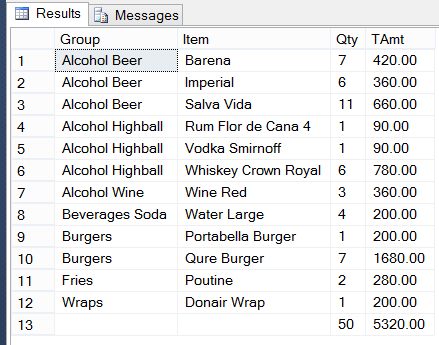3 Likes

what is the purpose of

just trying to understand the query.

I always do that. It has no functional purpose. It saves me having to comment out WHERE when I want to remove all conditions, or remove some but leave others in place. It lets me do this easily:

``````WHERE 1=1
and [CreatedDateTime] >= @dat_WorkPeriod_Beg
and [CreatedDateTime] <= @dat_WorkPeriod_End
-- and DecreaseInventory = 1 -- condition removed
and CalculatePrice <> 0
``````

i have run the query but still there is a slight difference in the report. here are the details:

sql data:

in query i set the date as: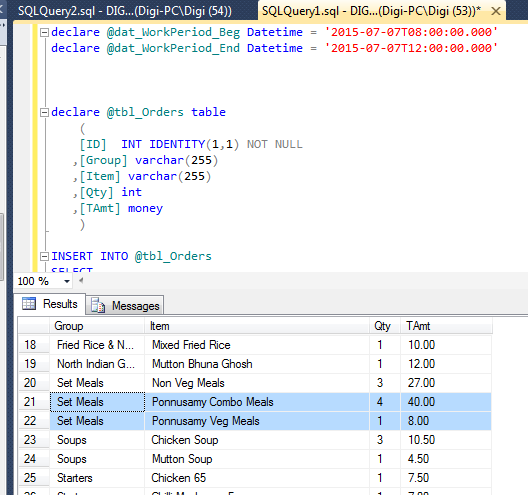and i get differences in qty where as the result is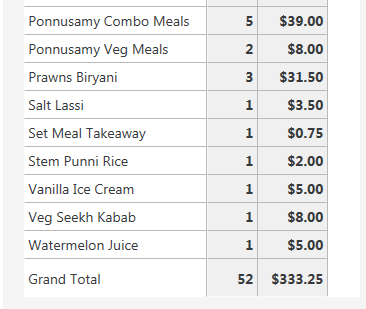and i get in sql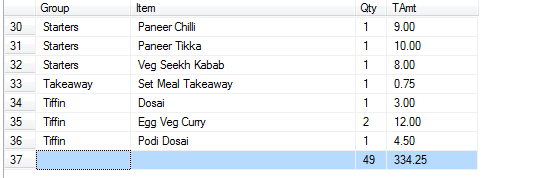I can’t tell anything by those screenshots. What exactly is wrong?

i have highlighted now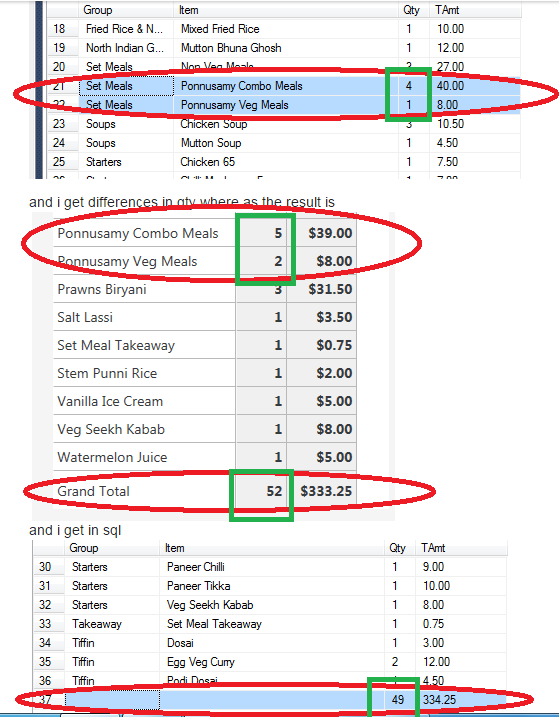Your report does not exclude voids (I guess) but QMcKay’s script does. Better create a new work period, make some sales, gifts, voids and after each transaction review how both reports changes. I think you’ll easily spot the difference.

i have checked with new work period and spotted the difference:

with SQL: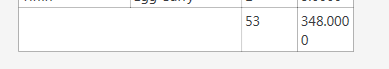if item voided ----- quantity decreases
if item given discount — original price stated
if item gifted ---- quantity decreases
with Pivot: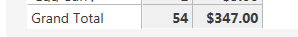if item voided ----- quanity doesnt decrease
if item given discount — price after discount stated

Your analysis of SQL is correct.

The [Orders] table is unaware of Discounts, unless the discount is given via an Order Tag. But in that case, you would need to parse the JSON data in the [OrderTags] field to determine the discount given by the specific tag(s), and subtract the value from the [Price] field. That is unless you are in the habit of adding the Order Tag Price to the Product Price, which may or may not be the case for you. Even if you add the Tag Price to the Order, the [Price] field will still contain the original Product Price.

You could also join the [Tickets] table along with the [AccountTransactions] table and the [AccountTransactionValues] table to determine effective price, but this is Ticket-based, not Order-based.

Either way, all of the above is rather complex, which is a good reason to use Report Tags instead.

It is up to you how you want to track Voids and Gifts via SQL. If you want to track Gifts, remove this:

`and [CalculatePrice] <> 0`

A similar thing could be said for `[DecreaseInventory]=1` … remove that condition to track Void [Qty].